• 进一步,针对其弱可观测性,采用多个被动传感器集中式融合跟踪策略,提出了基于无迹卡尔曼滤波的被动多传感器融合跟踪算法.以3个被动站跟踪为例进行仿真研究,结果表明所提出的算法可达到比经典的扩展卡尔曼滤波算法更高...
• ## 扩展卡尔曼滤波EKF与多传感器融合

万次阅读 多人点赞 2017-11-07 17:49:10
Extended Kalman Filter（扩展卡尔曼滤波）是卡尔曼滤波的非线性版本。在状态转移方程确定的情况下，EKF已经成为了非线性系统状态估计的事实标准。本文将简要介绍EKF，并介绍其在无人驾驶多传感器融合上的应用。
Extended Kalman Filter（扩展卡尔曼滤波）是卡尔曼滤波的非线性版本。在状态转移方程确定的情况下，EKF已经成为了非线性系统状态估计的事实标准。本文将简要介绍EKF，并介绍其在无人驾驶多传感器融合上的应用。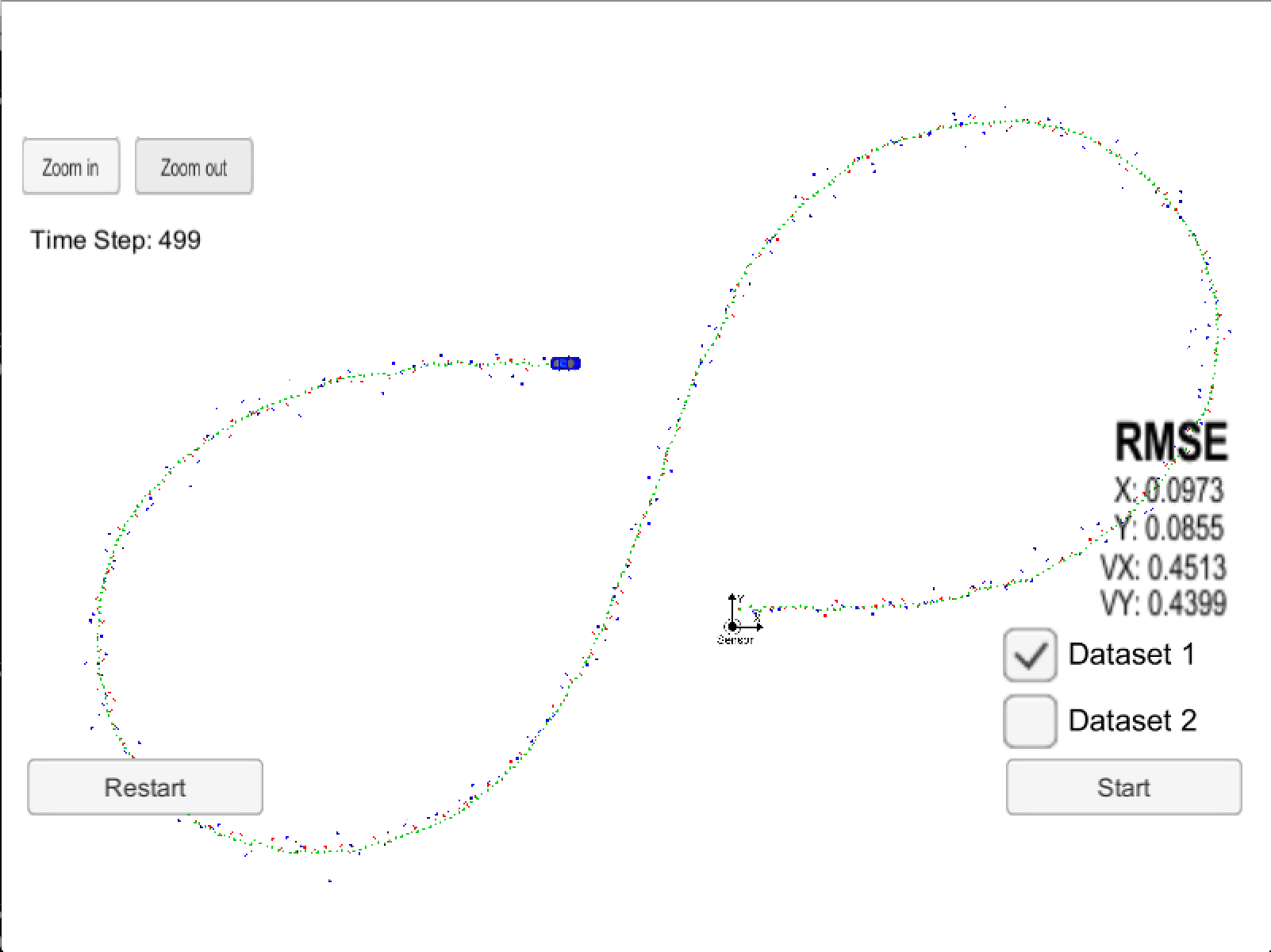KF与EKF
本文假定读者已熟悉KF，若不熟悉请参考卡尔曼滤波简介。
KF与EKF的区别如下：
预测未来：

x′=Fx+u
$x'=Fx+u$用

x′=f(x,u)
$x'=f(x,u)$代替；其余

F
$F$用Fj$F_j$代替。修正当下：将状态映射到测量的

Hx′
$Hx'$用

h(x′)
$h(x')$代替；其余

H
$H$用Hj$H_j$代替。
其中，非线性函数

f(x,u)，h(x′)
$f(x,u)，h(x')$用非线性得到了更精准的状态预测值、映射后的测量值；线性变换

Fj，Hj
$F_j，H_j$通过线性变换使得变换后的

x，z
$x，z$仍满足高斯分布的假设。

Fj，Hj
$F_j，H_j$计算方式如下：

Fjb=∂f(x,u)∂x=∂h(x′)∂x

\begin{split}
F_j &= \frac{\partial f(x, u)}{\partial x} \\
b &= \frac{\partial h(x')}{\partial x}
\end{split}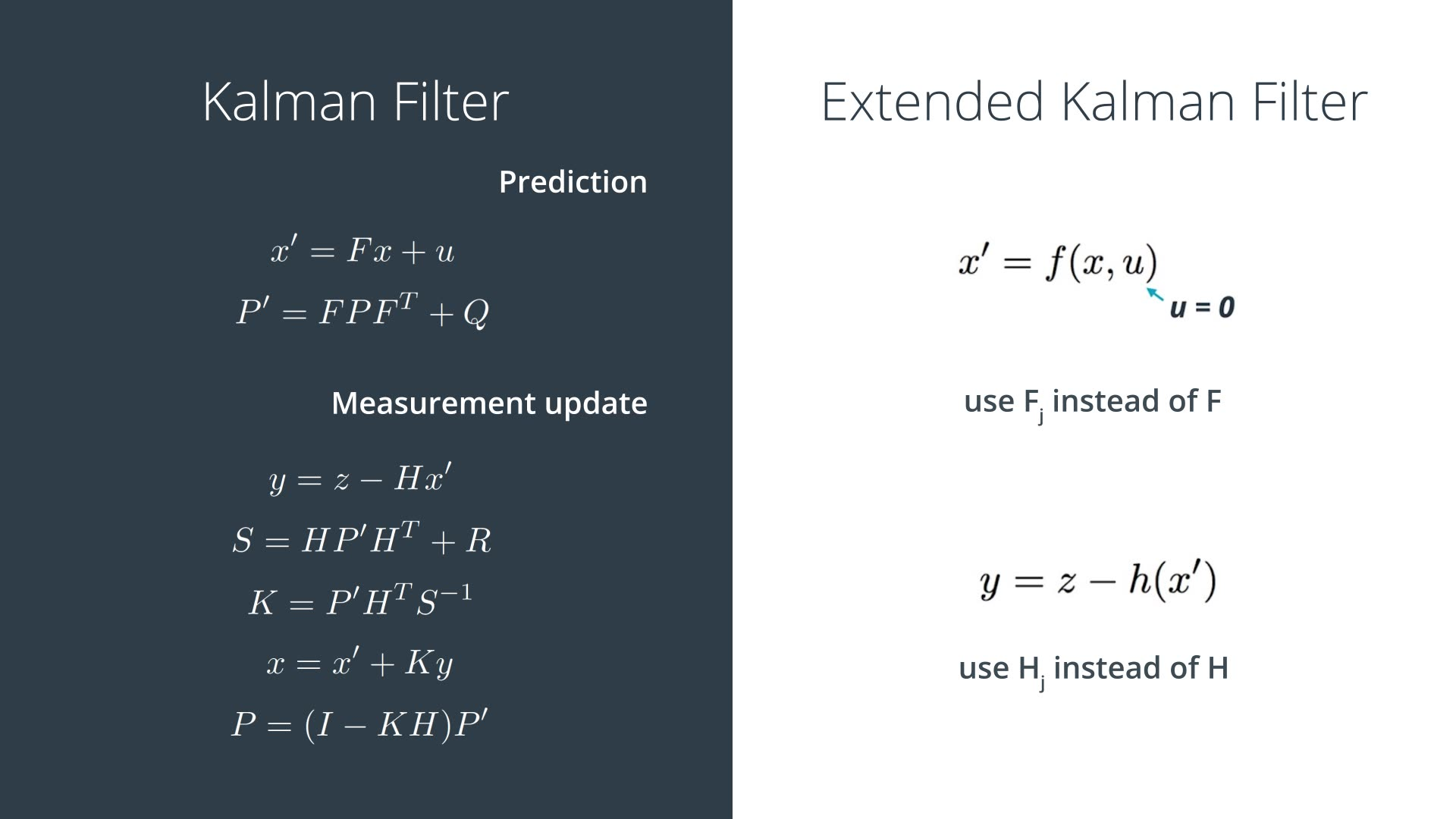为什么要用EKF
KF的假设之一就是高斯分布的

x
$x$预测后仍服从高斯分布，高斯分布的x$x$变换到测量空间后仍服从高斯分布。可是，假如

F、H
$F、H$是非线性变换，那么上述条件则不成立。
将非线性系统线性化
既然非线性系统不行，那么很自然的解决思路就是将非线性系统线性化。
对于一维系统，采用泰勒一阶展开即可得到：

f(x)≈f(μ)+∂f(μ)∂x(x−μ)

f(x) \approx f(\mu) + \frac{ \partial f(\mu)}{\partial x}(x-\mu)

对于多维系统，仍旧采用泰勒一阶展开即可得到：

T(x)≈f(a)+(x−a)TDf(a)

T(x) \approx f(a) + (x-a)^T Df(a)

其中，

Df(a)
$Df(a)$是Jacobian矩阵。
多传感器融合
本文将以汽车跟踪为例，目标是知道汽车时刻的状态

x=(px,py,vx,vy)
$x = (p_x, p_y, v_x, v_y)$。已知的传感器有lidar、radar。
lidar：笛卡尔坐标系。可检测到位置，没有速度信息。其测量值

z=(px,py)
$z=(p_x, p_y)$。radar：极坐标系。可检测到距离，角度，速度信息，但是精度较低。其测量值

z=(ρ,ϕ,ρ˙)
$z=(\rho, \phi, \dot{\rho})$，图示如下。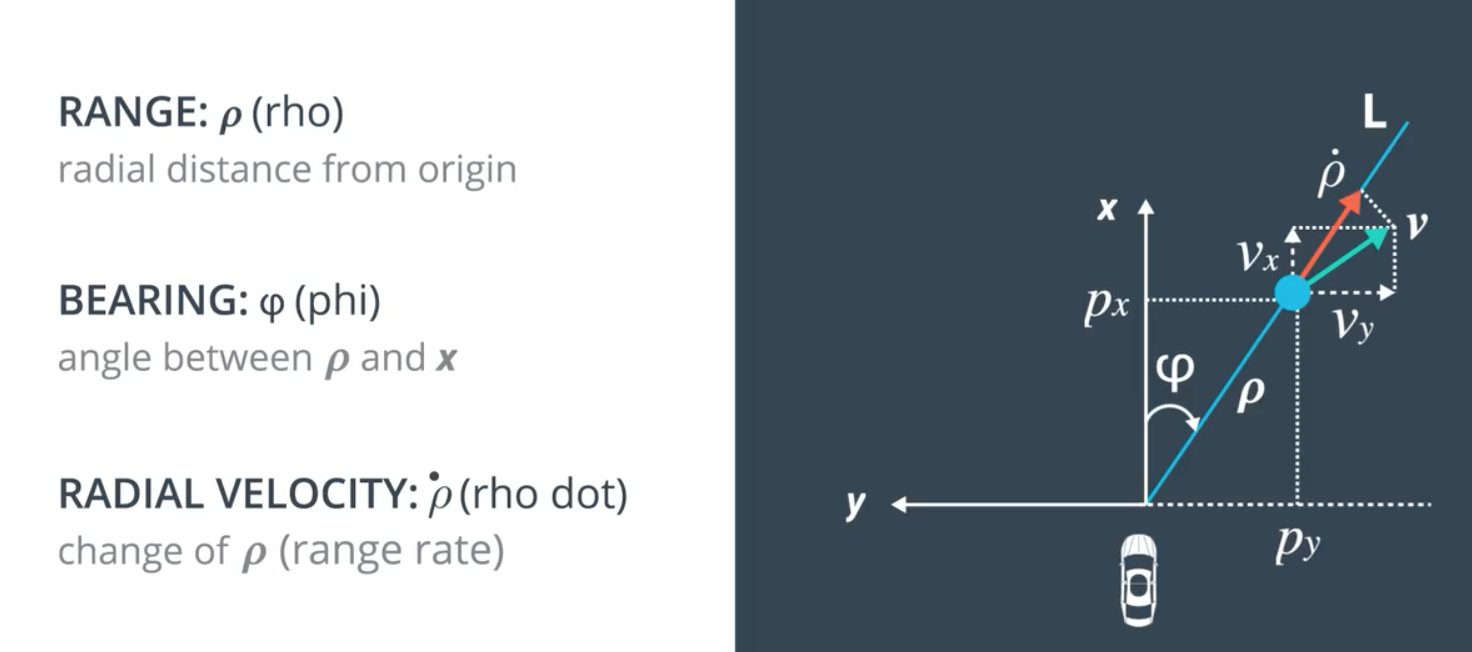传感器融合步骤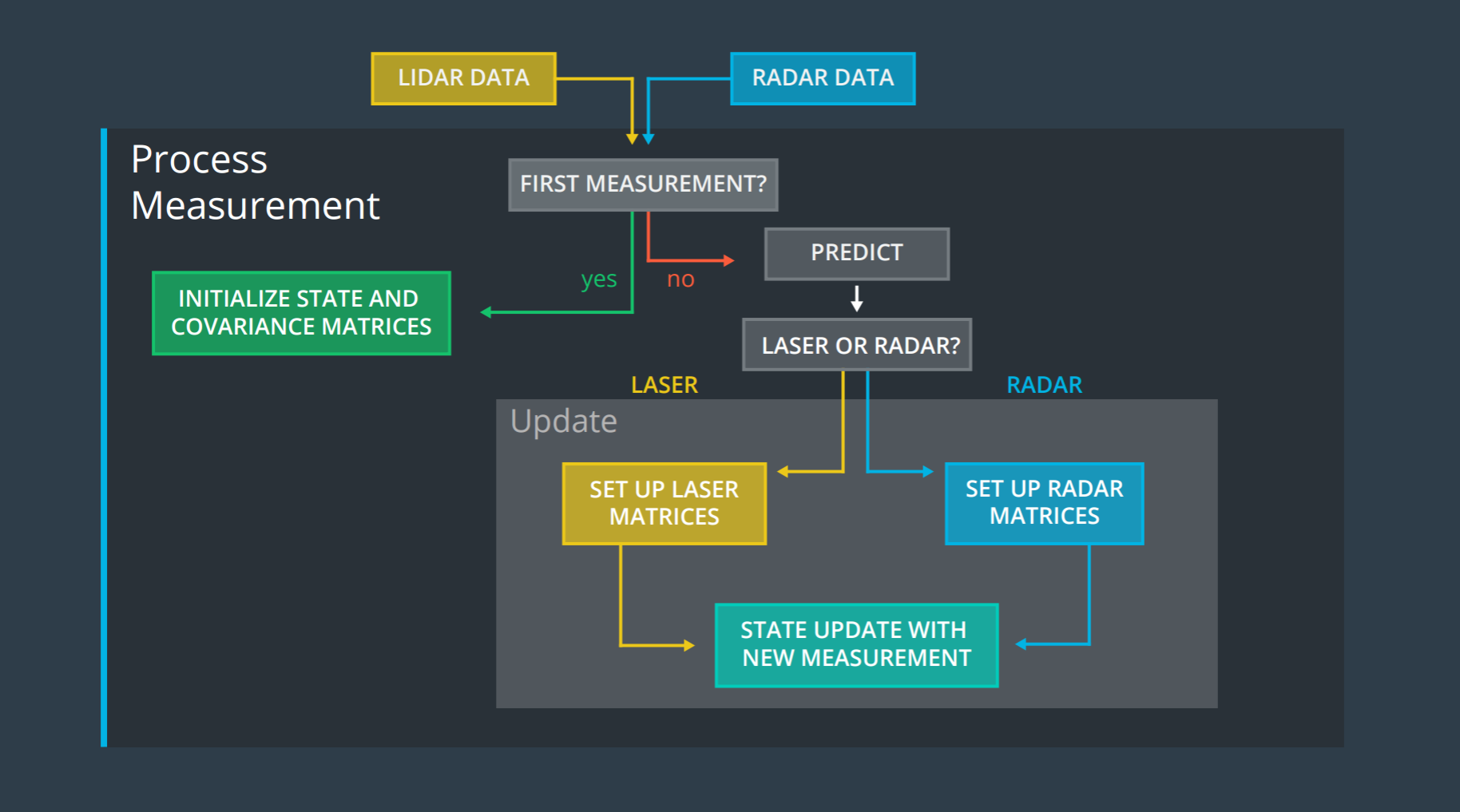步骤图如上所示，包括：
收到第一个测量值，对状态

x
$x$进行初始化。
预测未来
修正当下

初始化

初始化，指在收到第一个测量值后，对状态x$x$进行初始化。初始化如下，同时加上对时间的更新。 对于radar来说，

⎡⎣⎢⎢⎢⎢pxpyvxvy⎤⎦⎥⎥⎥⎥=⎡⎣⎢⎢⎢⎢10000100⎤⎦⎥⎥⎥⎥[pxpy]

\left[
\begin{matrix}
p_x \\
p_y \\
v_x \\
v_y
\end{matrix}
\right]
= \left[
\begin{matrix}
1 & 0 \\
0 & 1 \\
0 & 0 \\
0 & 0
\end{matrix}
\right]  \left[
\begin{matrix}
p_x \\
p_y
\end{matrix}

⎡⎣⎢⎢⎢⎢pxpyvxvy⎤⎦⎥⎥⎥⎥=⎡⎣⎢⎢⎢⎢ρcosϕρsinϕρ˙cosϕρ˙sinϕ⎤⎦⎥⎥⎥⎥

\left[
\begin{matrix}
p_x \\
p_y \\
v_x \\
v_y
\end{matrix}
\right]
= \left[
\begin{matrix}
\rho \cos{\phi} \\
\rho \sin{\phi} \\
\dot{\rho} \cos{\phi}\\
\dot{\rho} \sin{\phi}
\end{matrix}
\right]  预测未来 预测主要涉及的公式是：

x′P′=Fx=FPFT+Q

\begin{split}
x' &= Fx \\
P' &= FPF^T + Q
\end{split} 需要求解的有三个变量：

F、P、Q
$F、P、Q$。

F
$F$表明了系统的状态如何改变，这里仅考虑线性系统，F易得：

Fx=⎡⎣⎢⎢⎢⎢10000100dt0100dt01⎤⎦⎥⎥⎥⎥⎡⎣⎢⎢⎢⎢pxpyvxvy⎤⎦⎥⎥⎥⎥Fx = \left[
\begin{matrix}
1 & 0 & dt & 0 \\
0 & 1 & 0& dt \\
0 & 0 & 1& 0 \\
0 & 0 & 0& 1
\end{matrix}
\right]
\left[
\begin{matrix}
p_x \\
p_y \\
v_x \\
v_y
\end{matrix}
\right]

P
$P$表明了系统状态的不确定性程度，用x$x$的协方差表示，这里自己指定为：

P=⎡⎣⎢⎢⎢⎢1000010000100000001000⎤⎦⎥⎥⎥⎥

P = \left[
\begin{matrix}
1 & 0 & 0 & 0 \\
0 & 1 & 0& 0 \\
0 & 0 & 1000& 0 \\
0 & 0 & 0& 1000
\end{matrix}
\right]

Q
$Q$表明了x′=Fx$x' = Fx$未能刻画的其他外界干扰。本例子使用线性模型，因此加速度变成了干扰项。

x′=Fx
$x' = Fx$中未衡量的额外项目

v
$v$为：

v=⎡⎣⎢⎢⎢⎢⎢⎢⎢axdt22aydt22axdtaydt⎤⎦⎥⎥⎥⎥⎥⎥⎥=⎡⎣⎢⎢⎢⎢⎢⎢dt220dt00dt220dt⎤⎦⎥⎥⎥⎥⎥⎥[axay]=Gav = \left[
\begin{matrix}
\frac{a_x dt^2}{2} \\
\frac{a_y dt^2}{2} \\
a_x dt \\
a_y dt
\end{matrix}
\right] =
\left[
\begin{matrix}
\frac{dt^2}{2} & 0 \\
0 & \frac{dt^2}{2} \\
dt & 0 \\
0 & dt
\end{matrix}
\right]
\left[
\begin{matrix}
a_x\\
a_y
\end{matrix}
\right] = Ga

v
$v$服从高斯分布N(0,Q)$N(0, Q)$。

Q=E[vvT]=E[GaaTGT]=GE[aaT]GT=G[σ2ax00σ2ay]GT=⎡⎣⎢⎢⎢⎢⎢⎢⎢⎢⎢dt44σ2ax0dt32σ2ax00dt44σ2ay0dt32σ2aydt32σ2ax0dt2σ2ax00dt32σ2ay0dt2σ2ay⎤⎦⎥⎥⎥⎥⎥⎥⎥⎥⎥

\begin{split}
Q &= E[v v^T]= E[Gaa^TG^T] = GE[aa^T]G^T \\
&=  G\left[
\begin{matrix}
\sigma_{ax}^2 & 0\\
0 & \sigma_{ay}^2
\end{matrix}
\right] G^T \\
&=  \left[
\begin{matrix}
\frac{{dt}^4}{4} \sigma_{ax}^2 & 0 &  \frac{{dt}^3}{2} \sigma_{ax}^2  & 0 \\
0 &  \frac{{dt}^4}{4} \sigma_{ay}^2 & 0 & \frac{{dt}^3}{2} \sigma_{ay}^2 \\
\frac{{dt}^3}{2} \sigma_{ax}^2  & 0 & {dt}^2 \sigma_{ax}^2 & 0 \\
0 &    \frac{{dt}^3}{2} \sigma_{ay}^2  & 0& {dt}^2 \sigma_{ay}^2
\end{matrix}
\right]\end{split}  修正当下 lidar lidar使用了KF。修正当下这里牵涉到的公式主要是：

ySKx′P′=z−Hx=HPHT+R=PHTS−1=x+Ky=(I−KH)P

\begin{split}
y &= z - Hx \\
S &= HPH^T+R \\
K &= PH^TS^{-1} \\
x' &= x+Ky \\
P' &= (I-KH)P
\end{split} 需要求解的有两个变量：

H、R
$H、R$。

H
$H$表示了状态空间到测量空间的映射。

Hx=⎡⎣⎢⎢⎢⎢pxpyvxvy⎤⎦⎥⎥⎥⎥Hx = \left[
\begin{matrix}
1 & 0 & 0 & 0\\
0 & 1 & 0 & 0
\end{matrix}
\right]
\left[
\begin{matrix}
p_x \\
p_y \\
v_x \\
v_y
\end{matrix}
\right]

R
$R$表示了测量值的不确定度，一般由传感器的厂家提供，这里lidar参考如下：

Rlaser=[0.0225000.0225]R_{laser} = \left[
\begin{matrix}
0.0225 & 0  \\
0 & 0.0225
\end{matrix}

ySKx′P′=z−f(x)=HjPHTj+R=PHTjS−1=x+Ky=(I−KHj)P

\begin{split}
y &= z - f(x) \\
S &= H_jPH_j^T+R \\
K &= PH_j^TS^{-1} \\
x' &= x+Ky \\
P' &= (I-KH_j)P
\end{split} 区别与上面lidar的主要有：
状态空间到测量空间的非线性映射

f(x)
$f(x)$非线性映射线性化后的Jacob矩阵radar的

$R_{radar}$
状态空间到测量空间的非线性映射

f(x)
$f(x)$如下

f(x)=⎡⎣⎢⎢ρϕρ˙⎤⎦⎥⎥=⎡⎣⎢⎢⎢⎢⎢⎢⎢p2x+p2y‾‾‾‾‾‾‾√arctanpypxpxvx+pyvyp2x+p2y‾‾‾‾‾‾‾√⎤⎦⎥⎥⎥⎥⎥⎥⎥

f(x) = \left[
\begin{matrix}
\rho  \\
\phi \\
\dot{\rho}
\end{matrix}
\right] =
\left[
\begin{matrix}
\sqrt{p_x^2+p_y^2}  \\
\arctan{\frac{p_y}{p_x}} \\
\frac{p_x v_x + p_y v_y}{\sqrt{p_x^2+p_y^2}  }
\end{matrix}\right]
非线性映射线性化后的Jacob矩阵

Hj
$H_j$

Hj=∂f(x)∂x=⎡⎣⎢⎢⎢⎢⎢⎢⎢∂ρ∂px∂ϕ∂px∂ρ˙∂px∂ρ∂py∂ϕ∂py∂ρ˙∂py∂ρ∂vx∂ϕ∂vx∂ρ˙∂vx∂ρ∂vy∂ϕ∂vy∂ρ˙∂vy⎤⎦⎥⎥⎥⎥⎥⎥⎥

H_j = \frac{\partial f(x)}{\partial x} =
\left[
\begin{matrix}
\frac{\partial \rho}{\partial p_x} & \frac{\partial \rho}{\partial p_y} & \frac{\partial \rho}{\partial v_x} & \frac{\partial \rho}{\partial v_y}  \\
\frac{\partial \phi}{\partial p_x} & \frac{\partial \phi}{\partial p_y} & \frac{\partial \phi}{\partial v_x} & \frac{\partial \phi}{\partial v_y}  \\
\frac{\partial \dot{\rho}}{\partial p_x} & \frac{\partial \dot{\rho}}{\partial p_y} & \frac{\partial \dot{\rho}}{\partial v_x} & \frac{\partial \dot{\rho}}{\partial v_y}
\end{matrix}
\right]

R
$R$表示了测量值的不确定度，一般由传感器的厂家提供，这里radar参考如下：

Rlaser=⎡⎣⎢⎢0.090000.00090000.09⎤⎦⎥⎥R_{laser} = \left[
\begin{matrix}
0.09 & 0  & 0\\
0 & 0.0009 & 0 \\
0 & 0 & 0.09
\end{matrix}
\right] 传感器融合实例 多传感器融合的示例如下，需要注意的有：
lidar和radar的预测部分是完全相同的lidar和radar的参数更新部分是不同的，不同的原因是不同传感器收到的测量值是不同的当收到lidar或radar的测量值，依次执行预测、更新步骤当同时收到lidar和radar的测量值，依次执行预测、更新1、更新2步骤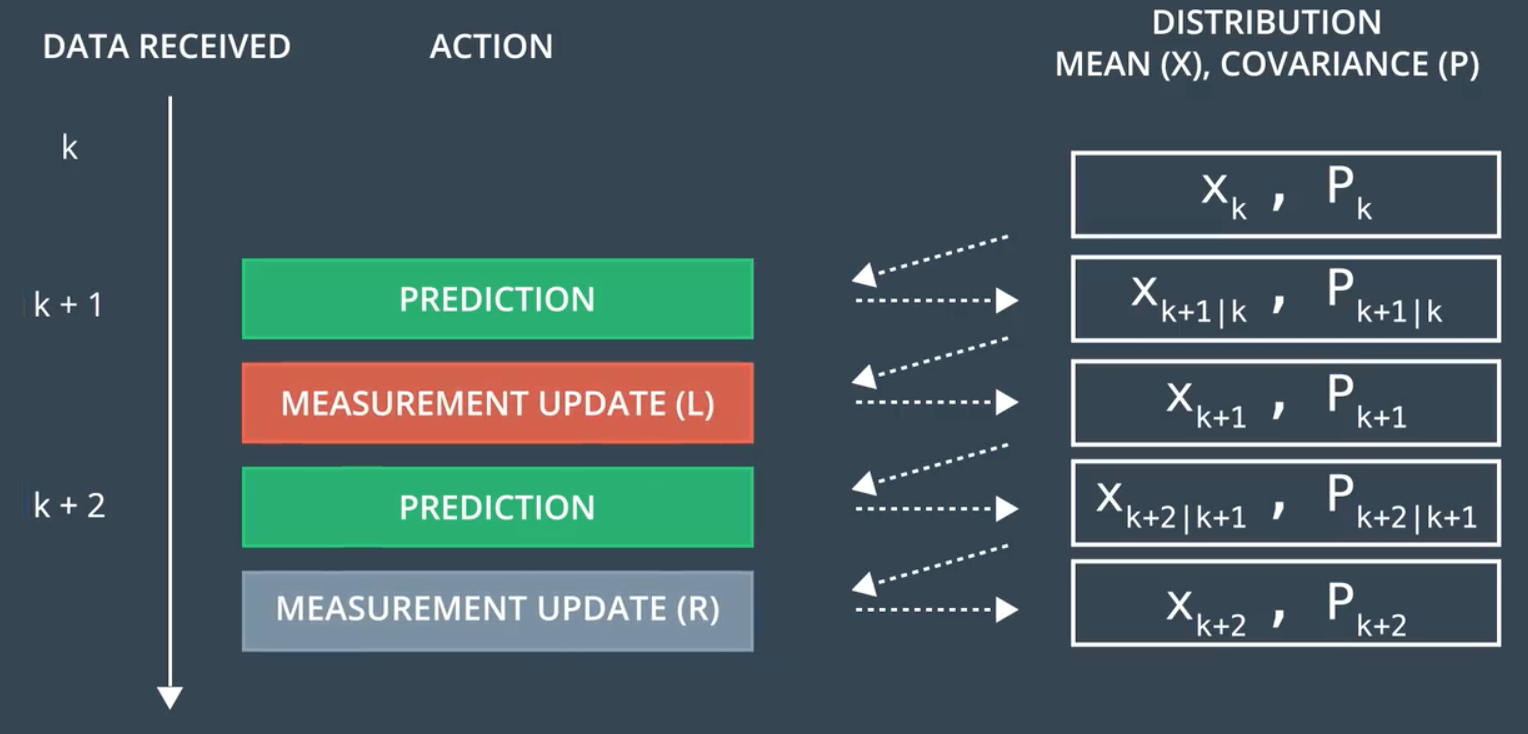多传感器融合的效果如下图所示，红点和蓝点分别表示radar和lidar的测量位置，绿点代表了EKF经过多传感器融合后获取到的测量位置，取得了较低的RMSE。EKF
• 首先基于周期性分组传输通信策略,采用序贯卡尔曼滤波方法,对当前时刻访问融合中心的传感器组进行局部滤波,并导出剩余传感器组的最优局部估计,进而得到线性最小方差意义下的最优融合估计.利用残差加权平方和方法对...
• ## 详解卡尔曼滤波原理

万次阅读 多人点赞 2017-03-18 13:54:15
详解卡尔曼滤波原理　在网上看了不少与卡尔曼滤波相关的博客、论文，要么是只谈理论、缺乏感性，或者有感性认识，缺乏理论推导。能兼顾二者的少之又少，直到我看到了国外的一篇博文，真的惊艳到我了，不得不佩服作者...


详解卡尔曼滤波原理

在网上看了不少与卡尔曼滤波相关的博客、论文，要么是只谈理论、缺乏感性，或者有感性认识，缺乏理论推导。能兼顾二者的少之又少，直到我看到了国外的一篇博文，真的惊艳到我了，不得不佩服作者这种细致入微的精神，翻译过来跟大家分享一下，原文链接：http://www.bzarg.com/p/how-a-kalman-filter-works-in-pictures/  　　我不得不说说卡尔曼滤波，因为它能做到的事情简直让人惊叹！意外的是很少有软件工程师和科学家对对它有所了解，这让我感到沮丧，因为卡尔曼滤波是一个如此强大的工具，能够在不确定性中融合信息，与此同时，它提取精确信息的能力看起来不可思议。
什么是卡尔曼滤波？
我们能用卡尔曼滤波做什么？
用玩具举例：你开发了一个可以在树林里到处跑的小机器人，这个机器人需要知道它所在的确切位置才能导航。我们可以说机器人有一个状态 ，表示位置和速度：

注意这个状态只是关于这个系统基本属性的一堆数字，它可以是任何其它的东西。在这个例子中是位置和速度，它也可以是一个容器中液体的总量，汽车发动机的温度，用户手指在触摸板上的位置坐标，或者任何你需要跟踪的信号。  　　这个机器人带有GPS，精度大约为10米，还算不错，但是，它需要将自己的位置精确到10米以内。树林里有很多沟壑和悬崖，如果机器人走错了一步，就有可能掉下悬崖，所以只有GPS是不够的。或许我们知道一些机器人如何运动的信息：例如，机器人知道发送给电机的指令，知道自己是否在朝一个方向移动并且没有人干预，在下一个状态，机器人很可能朝着相同的方向移动。当然，机器人对自己的运动是一无所知的：它可能受到风吹的影响，轮子方向偏了一点，或者遇到不平的地面而翻倒。所以，轮子转过的长度并不能精确表示机器人实际行走的距离，预测也不是很完美。  　　GPS 传感器告诉了我们一些状态信息，我们的预测告诉了我们机器人会怎样运动，但都只是间接的，并且伴随着一些不确定和不准确性。但是，如果使用所有对我们可用的信息，我们能得到一个比任何依据自身估计更好的结果吗？回答当然是YES，这就是卡尔曼滤波的用处。
卡尔曼滤波是如何看到你的问题的
下面我们继续以只有位置和速度这两个状态的简单例子做解释。

我们并不知道实际的位置和速度，它们之间有很多种可能正确的组合，但其中一些的可能性要大于其它部分：卡尔曼滤波假设两个变量（位置和速度，在这个例子中）都是随机的，并且服从高斯分布。每个变量都有一个均值 μ，表示随机分布的中心（最可能的状态），以及方差 ，表示不确定性。在上图中，位置和速度是不相关的，这意味着由其中一个变量的状态无法推测出另一个变量可能的值。下面的例子更有趣：位置和速度是相关的，观测特定位置的可能性取决于当前的速度：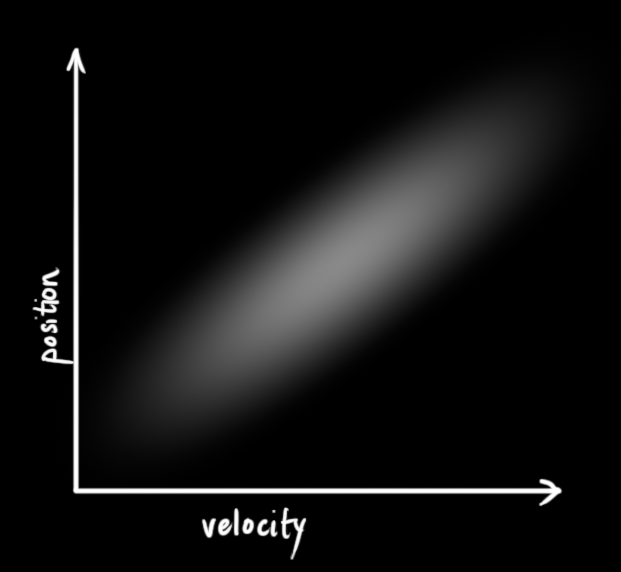这种情况是有可能发生的，例如，我们基于旧的位置来估计新位置。如果速度过高，我们可能已经移动很远了。如果缓慢移动，则距离不会很远。跟踪这种关系是非常重要的，因为它带给我们更多的信息：其中一个测量值告诉了我们其它变量可能的值，这就是卡尔曼滤波的目的，尽可能地在包含不确定性的测量数据中提取更多信息！  　　这种相关性用协方差矩阵来表示，简而言之，矩阵中的每个元素  表示第 i 个和第 j 个状态变量之间的相关度。（你可能已经猜到协方差矩阵是一个对称矩阵，这意味着可以任意交换 i 和 j）。协方差矩阵通常用“”来表示，其中的元素则表示为“ ”。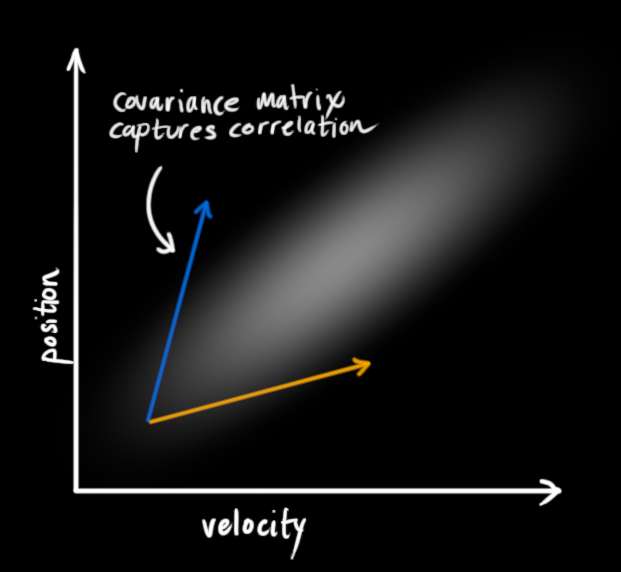使用矩阵来描述问题
我们基于高斯分布来建立状态变量，所以在时刻 k 需要两个信息：最佳估计 （即均值，其它地方常用 μ 表示），以及协方差矩阵  。

(1)

（当然，在这里我们只用到了位置和速度，实际上这个状态可以包含多个变量，代表任何你想表示的信息）。接下来，我们需要根据当前状态（k-1 时刻）来预测下一状态（k 时刻）。记住，我们并不知道对下一状态的所有预测中哪个是“真实”的，但我们的预测函数并不在乎。它对所有的可能性进行预测，并给出新的高斯分布。我们可以用矩阵  来表示这个预测过程：它将我们原始估计中的每个点都移动到了一个新的预测位置，如果原始估计是正确的话，这个新的预测位置就是系统下一步会移动到的位置。那我们又如何用矩阵来预测下一个时刻的位置和速度呢？下面用一个基本的运动学公式来表示：现在，我们有了一个预测矩阵来表示下一时刻的状态，但是，我们仍然不知道怎么更新协方差矩阵。此时，我们需要引入另一个公式，如果我们将分布中的每个点都乘以矩阵 A，那么它的协方差矩阵  会怎样变化呢？很简单，下面给出公式：结合方程（4）和（3）得到：外部控制量
我们并没有捕捉到一切信息，可能存在外部因素会对系统进行控制，带来一些与系统自身状态没有相关性的改变。  　　以火车的运动状态模型为例，火车司机可能会操纵油门，让火车加速。相同地，在我们机器人这个例子中，导航软件可能会发出一个指令让轮子转向或者停止。如果知道这些额外的信息，我们可以用一个向量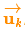来表示，将它加到我们的预测方程中做修正。  　　假设由于油门的设置或控制命令，我们知道了期望的加速度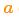，根据基本的运动学方程可以得到：以矩阵的形式表示就是：称为控制矩阵，称为控制向量（对于没有外部控制的简单系统来说，这部分可以忽略）。让我们再思考一下，如果我们的预测并不是100%准确的，该怎么办呢？
外部干扰
如果这些状态量是基于系统自身的属性或者已知的外部控制作用来变化的，则不会出现什么问题。  　　但是，如果存在未知的干扰呢？例如，假设我们跟踪一个四旋翼飞行器，它可能会受到风的干扰，如果我们跟踪一个轮式机器人，轮子可能会打滑，或者路面上的小坡会让它减速。这样的话我们就不能继续对这些状态进行跟踪，如果没有把这些外部干扰考虑在内，我们的预测就会出现偏差。  　　在每次预测之后，我们可以添加一些新的不确定性来建立这种与“外界”（即我们没有跟踪的干扰）之间的不确定性模型：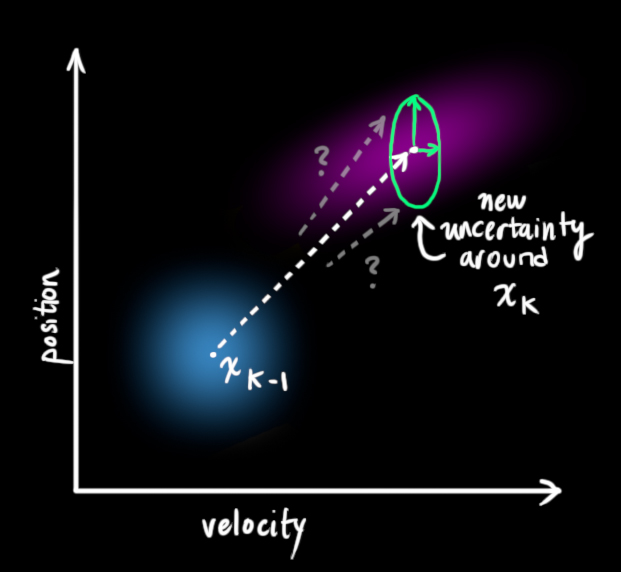原始估计中的每个状态变量更新到新的状态后，仍然服从高斯分布。我们可以说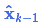的每个状态变量移动到了一个新的服从高斯分布的区域，协方差为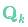。换句话说就是，我们将这些没有被跟踪的干扰当作协方差为的噪声来处理。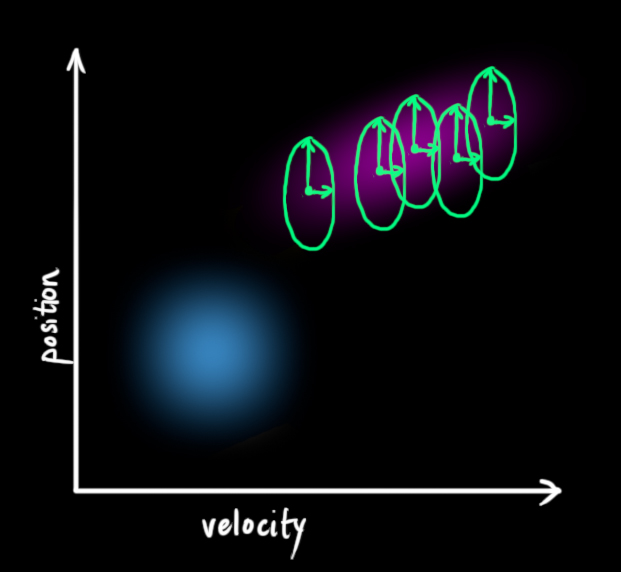这产生了具有不同协方差（但是具有相同的均值）的新的高斯分布。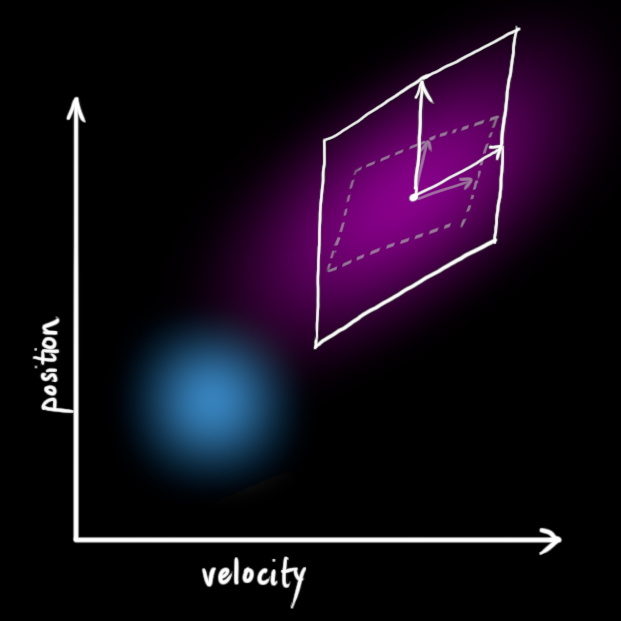我们通过简单地添加得到扩展的协方差，下面给出预测步骤的完整表达式：由上式可知，新的最优估计是根据上一最优估计预测得到的，并加上已知外部控制量的修正。  　　而新的不确定性由上一不确定性预测得到，并加上外部环境的干扰。  　　好了，我们对系统可能的动向有了一个模糊的估计，用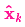和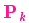来表示。如果再结合传感器的数据会怎样呢？
用测量值来修正估计值
我们可能会有多个传感器来测量系统当前的状态，哪个传感器具体测量的是哪个状态变量并不重要，也许一个是测量位置，一个是测量速度，每个传感器间接地告诉了我们一些状态信息。注意，传感器读取的数据的单位和尺度有可能与我们要跟踪的状态的单位和尺度不一样，我们用矩阵  来表示传感器的数据。我们可以计算出传感器读数的分布，用之前的表示方法如下式所示：卡尔曼滤波的一大优点就是能处理传感器噪声，换句话说，我们的传感器或多或少都有点不可靠，并且原始估计中的每个状态可以和一定范围内的传感器读数对应起来。从测量到的传感器数据中，我们大致能猜到系统当前处于什么状态。但是由于存在不确定性，某些状态可能比我们得到的读数更接近真实状态。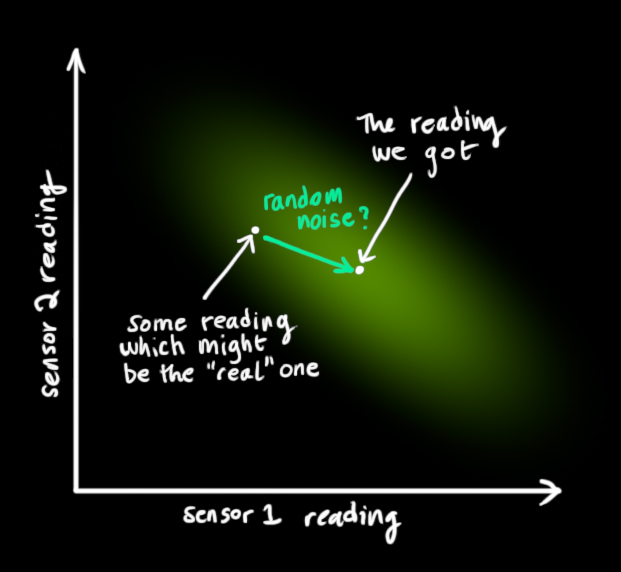我们将这种不确定性（例如：传感器噪声）用协方差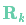表示，该分布的均值就是我们读取到的传感器数据，称之为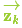。  现在我们有了两个高斯分布，一个是在预测值附近，一个是在传感器读数附近。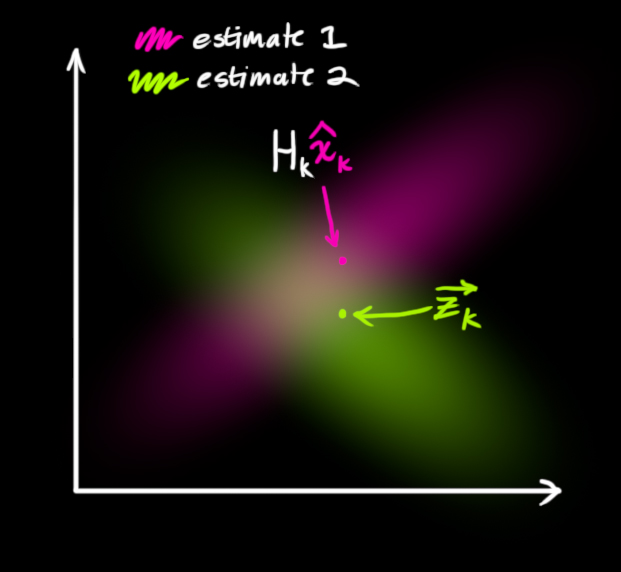我们必须在预测值（粉红色）和传感器测量值（绿色）之间找到最优解。  　　那么，我们最有可能的状态是什么呢？对于任何可能的读数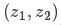，有两种情况：（1）传感器的测量值；（2）由前一状态得到的预测值。如果我们想知道这两种情况都可能发生的概率，将这两个高斯分布相乘就可以了。剩下的就是重叠部分了，这个重叠部分的均值就是两个估计最可能的值，也就是给定的所有信息中的最优估计。  　　瞧！这个重叠的区域看起来像另一个高斯分布。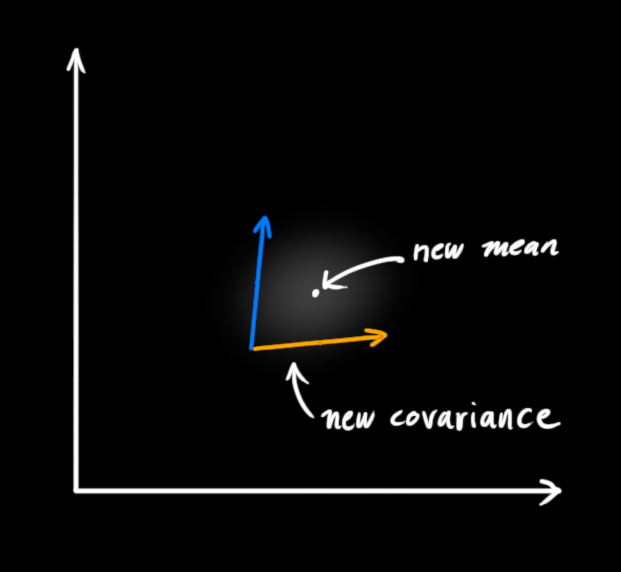如你所见，把两个具有不同均值和方差的高斯分布相乘，你会得到一个新的具有独立均值和方差的高斯分布！下面用公式讲解。
融合高斯分布
先以一维高斯分布来分析比较简单点，具有方差  和 μ 的高斯曲线可以用下式表示：如果把两个服从高斯分布的函数相乘会得到什么呢？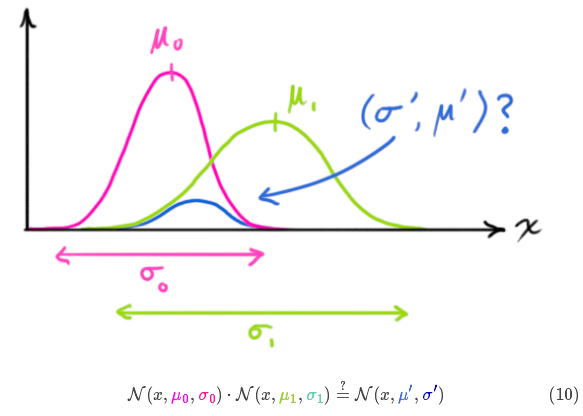将式（9）代入到式（10）中（注意重新归一化，使总概率为1）可以得到：将式（11）中的两个式子相同的部分用 k 表示：下面进一步将式（12）和（13）写成矩阵的形式，如果 Σ 表示高斯分布的协方差， 表示每个维度的均值，则：矩阵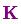称为卡尔曼增益，下面将会用到。放松！我们快要完成了！
将所有公式整合起来
我们有两个高斯分布，预测部分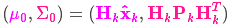，和测量部分，将它们放到式（15）中算出它们之间的重叠部分：由式（14）可得卡尔曼增益为：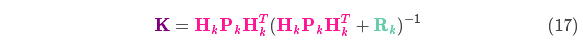将式（16）和式（17）的两边同时左乘矩阵的逆（注意里面包含了  ）将其约掉，再将式（16）的第二个等式两边同时右乘矩阵  的逆得到以下等式：上式给出了完整的更新步骤方程。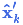就是新的最优估计，我们可以将它和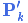放到下一个预测和更新方程中不断迭代。总结
以上所有公式中，你只需要用到式（7）、（18）、（19）。（如果忘了的话，你可以根据式（4）和（15）重新推导一下）  　　我们可以用这些公式对任何线性系统建立精确的模型，对于非线性系统来说，我们使用扩展卡尔曼滤波，区别在于EKF多了一个把预测和测量部分进行线性化的过程。
（ps: 第一次用Markdown，添加图片和公式心累啊，什么时候能直接拖拽就好了~~）
附Markdown使用技巧：  1. 改变文本字体、字号与颜色。参考链接：(http://blog.csdn.net/testcs_dn/article/details/45719357/)  2. 在线公式编辑器，编辑好了右键“复制图片地址”，当作图片来添加。  　链接：(http://private.codecogs.com/latex/eqneditor.php)  3. 段落首行缩进，按Shift+Space将输入法切换到全角状态，然后敲空格即可，一个空格代表一个汉字的间隔。  4. 设置图片大小及居中显示。参考链接：(http://blog.csdn.net/soindy/article/details/50427079)  5. 不懂百度。
展开全文• ## 卡尔曼滤波数据融合算法

万次阅读 多人点赞 2017-11-10 14:06:43
卡尔曼滤波数据融合算法 小狼@http://blog.csdn.net/xiaolangyangyang


卡尔曼滤波数据融合算法
小狼@http://blog.csdn.net/xiaolangyangyang

谈谈MPU6050的数据融合 一阶滤波 卡尔曼滤波

如何通俗并尽可能详细解释卡尔曼滤波？

[ROS] 多传感器卡尔曼融合框架 Ethzasl MSF Framework 编译与使用

多源数据融合：大数据分析的瓶颈

03-数据融合-ROS轮式机器人数据融合-odom&IMU

C语言实现卡尔曼滤波如何设定噪声?

[经验] 教你如何用C语言实现卡尔曼滤波

卡尔曼滤波算法及C语言实现

如何通俗易懂地解释「协方差」与「相关系数」的概念？

融合imu的rgbd-slam算法改进研究

协方差矩阵怎么求

Orocos Bayesian Filtering Library

什么是机器人定位robot_localization

Bayes++ Library入门学习之熟悉class-Bayesian_filter_base(1)

能否简单并且易懂地介绍一下多个基于滤波方法的SLAM算法原理？

卡尔曼滤波器是如何运用于多传感器融合的？

卡尔曼滤波C代码分析

GD32F103+MPU9150四旋翼飞行器第一步：姿态融合算法

单目/双目与imu的融合（一）

关于stm32进行卡尔曼滤波制作平衡车的一些心得和理解


展开全文• （一）卡尔曼滤波的原理 关于卡尔曼滤波的原理，可以参考Bzarg所写的《How a Kalman filter works, in pictures》，卡尔曼滤波总体框图如下所示。 我自己总结卡尔曼滤波主要有以下两个步骤： 1）根据估算值...
（一）卡尔曼滤波的原理
关于卡尔曼滤波的原理，可以参考Bzarg所写的《How a Kalman filter works, in pictures》，卡尔曼滤波总体框图如下所示。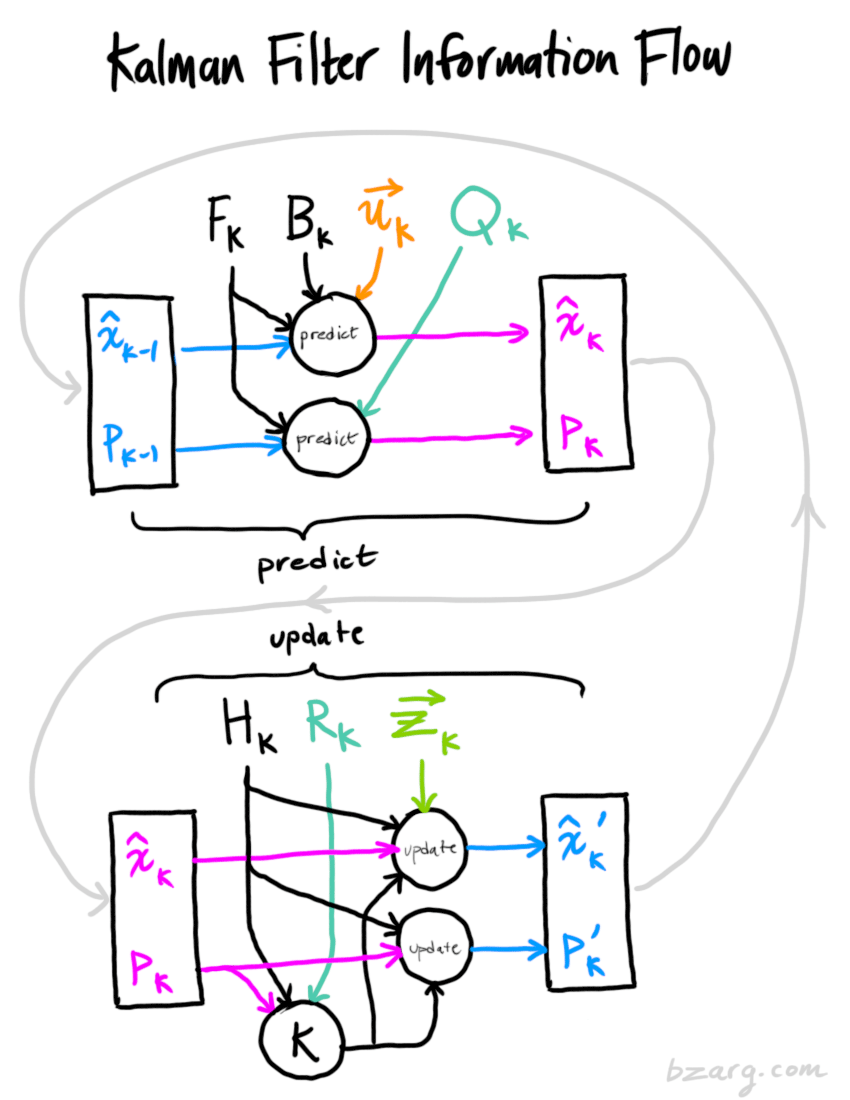我自己总结卡尔曼滤波主要有以下两个步骤：
1）根据估算值得到预测值；
2）根据测量值对预测值进行更新，得到新的估算值。
（二）GPS和惯性传感器IMU的融合
GPS的缺点是更新频率低，无法满足实时性计算的要求。惯性传感器IMU是高频传感器，在短时间内可以提供稳定的实时位置更新，但是其定位误差随着运行时间增加。所以需要融合这两种传感器的优点，才能得到实时和精准的定位。
如下图所示，使用卡尔曼滤波器对惯性传感器IMU与GPS数据进行融合。首先，我们在上一次位置估算的基础上使用惯性传感器IMU对当前的位置进行实时预测。在得到新的GPS数据之前，我们只能通过积分惯性传感器IMU的数据预测当前位置。惯性传感器IMU的定位误差会随着运行时间增长，所以当接收到新的GPS数据时，由于GPS的数据比较精准，我们可以使用GPS数据对当前的位置预测进行更新。通过不断地执行这两个步骤，可以取两者所长，对无人车进行准确的实时定位。假设惯性传感器的频率是1kHz，而GPS的频率是10Hz，那么每两次GPS更新之间会使用100个惯性传感器数据点进行位置预测。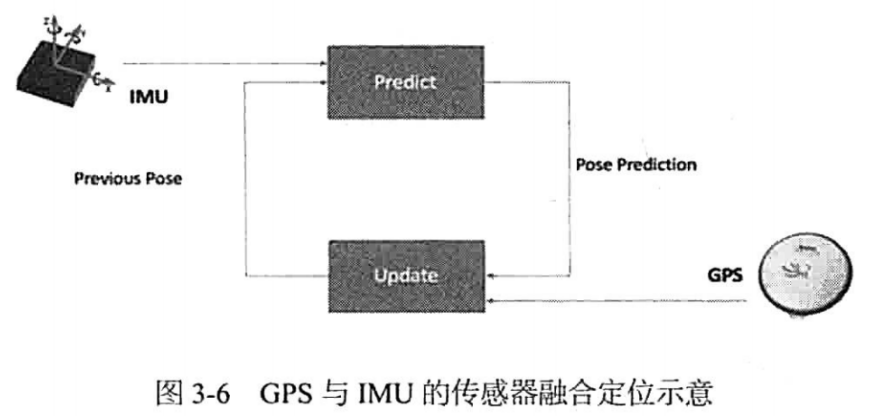参考：
1）How a Kalman filter works, in pictures，Bzarg
2）第一本无人驾驶技术书，刘少山等著，北京：电子工业出版社，2017.6


展开全文• 针对目前国内市场智能钓鱼竿功能不足的问题，本文通过四元数法对鱼竿姿态解算，利用基于卡尔曼滤波的数据融合算法，并以微处理器STM32为核心搭建uC/OS-II操作系统，结合多种环境传感器和串级PID控制方法，设计了一种...
• 进一步，针对其弱可观测性，采用多个被动传感器集中式融合跟踪策略，提出了基于无迹卡尔曼滤波的被动多传感器融合跟踪算法．以3个被动站跟踪为例进行仿真研究，结果表明所提出的算法可达到比经典的扩展卡尔曼滤波...
• 卡尔曼滤波的缺点就是需要精确的模型，所以由此产生了自校正卡尔曼滤波 自适应卡尔曼滤波 所以都是在解决问题中产生 下面拍自《多传感器加权观测融合kalman滤波理论》 下面拍自《现代信息融合技术在组合...
• 一文理清卡尔曼滤波，从传感器数据融合开始谈起 5/19,'19 FesianXu 前言 最近在一个项目中接触到了卡尔曼滤波，并且对此进行了学习，发现其是一个很有意思的信息融合的算法，可以结合多种传感器的信息（存在噪声...
• 在信息融合中经常使用卡尔曼滤波，现在我们对其进行讲解： 百度百科上写到： 卡尔曼滤波公式如上 这是另一种表述，涉及的符号见下表： 对于联邦卡尔曼滤波： 对于一致性卡尔曼滤波： ...信号处理
• 这是有优达学城的无人驾驶纳米学位第五个项目； 1. 传感器 在无人驾驶系统中，对物体跟踪和预测，目前常用...如果能够把激光和雷达的测量值都融合卡尔曼滤波系统中，那么可以更好的改进跟踪系统，能够准确地估算行...自动驾驶
• 在集中式滤波结构和联邦式滤波结构的基础上，设计了一种基于自适应容积卡尔曼滤波算法的多传感器系统混合式组合滤波结构，并给出了融合传感器的局部滤波信息以得到全局滤波估计的计算方法。以对车辆的定位导航为...自适应 组合滤波
• 在集中式滤波结构和联邦式滤波结构的基础上，设计了一种基于自适应容积卡尔曼滤波算法的多传感器系统混合式组合滤波结构，并给出了融合传感器的局部滤波信息以得到全局滤波估计的计算方法。以对车辆的定位导航为...
• MPU6050_Kalman_PWM_remote 基于卡尔曼滤波的多传感器测量数据融合 MPU6050_卡尔曼滤波 KF算法流程 自平衡小车 双轮自平衡小车设计报告 陀螺仪+加速度计卡尔曼
• 由于研究需要，最近在看卡尔曼滤波，做个小总结。 最佳线性滤波理论起源于40年代美国科学家Wiener和前苏联科学家Kолмогоров等人的研究工作，后人统称为维纳滤波理论。从理论上说，维纳滤波的最大缺点是必须...
• 工程实践证明, 标准卡尔曼滤波器的鲁棒性较差.... 针对此问题, 提出了基于自适应衰减卡尔曼滤波的多传感器信息融合方法, 这种方法可以有效地消除系统状态方程在建模存在误差时给信息融合带来的影响.
• Extended Kalman Filter（扩展卡尔曼滤波）是卡尔曼滤波的非线性版本。在状态转移方程确定的情况下，EKF已经成为了非线性系统状态估计的事实标准。本文将简要介绍EKF，并介绍其在无人驾驶多传感器融合上的应用。 ...Kalman
• 车辆稳定性控制系统能够提高...对于质心侧偏角的估算国内外学者也有进行不同程度的探索，但是大多采用的是积分算法、卡尔曼滤波算法等等，但是在工程实际应用中却很少。 质心侧偏角通常定义为侧向车速与纵向车速的...算法
• 最近需要做一个空中飞鼠（AirMouse）项目，其中对六轴陀螺仪回传的...其中我着重学习了卡尔曼滤波和扩展卡尔曼滤波，并对扩展卡尔曼滤波进行实现。 1. 卡尔曼滤波学习 卡尔曼滤波算法是卡尔曼（Kalman）等人在20世纪EKF matlab
• 本文针对运动检测中的速度传感器与加速度传感器，给出了一种应用卡尔曼滤波原理对多传感器进行数据融合的方法，得到了该方法下滤波器状态矩阵和相关矩阵的一般表达式，仿真与试验证明，运用该方法可以得到很好的滤波...
• 多尺度无味卡尔曼滤波方法的自适应融合设计用于多传感器数据融合研究论文
• 基于卡尔曼滤波的陀螺仪/地磁传感器融合测姿研究，李岩，邱海迪，为提高滑翔增程制导炮弹的长时远程姿态测量精度，降低惯性陀螺姿态测量零漂的影响，研究了基于陀螺和地磁传感器融合测量弹体姿态
• 卡尔曼滤波与航迹融合 文章目录卡尔曼滤波与航迹融合前言一、航迹数据仿真二、卡尔曼滤波器1.举例说明2.卡尔曼滤波算法.总结 前言 这是我雷达信号处理课的一个作业,有些知识我也是新学的,原理也不是很懂. 所谓的...算法
• 背景介绍 卡尔曼滤波（Kalman filtering）...目前在机器人导航，控制，传感器数据融合甚至在军事方面的雷达系统以及导弹追踪等等应用较广。近年来更被应用于计算机图像处理，例如头脸识别，图像分割，图像边缘检测...
•空空如也空空如也

...
收藏数 2,640
精华内容 1,056
热门标签
关键字：